# UNFOLD

This operator was also proposed in Gyssens & Lakshamanan (1997). Analogous to the previous operator, let s be a MAD with schema S, let d be a new name of the set of names N appearing nowhere else in s, and let X M be a set of measure attributes (it is important to note that, defining M as R - 1in Xi, the authors highlight the uniform or symmetric treatment of category attributes and measures).

We define unfolddX(s) as a table with schema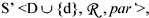where, for all di in D, par' (di) = par (di) and par' (d) = X and with instance tabS' (rep(s)).

### Relate Levels

This operator, like the following three operators, has been proposed in Hurtado, Mendelzon, & Vaisman (1999).

The relate levels operator defines a roll-up function between two independent levels belonging to a dimension. A necessary condition for this is the existence of a function f between the instance sets of the levels being related, such that the dimension instance remains consistent. Otherwise, the relate levels cannot be applied. Moreover, when conditions for relating two levels la and lb are met, we must delete all the redundant roll-up functions that may appear, which are not admitted in the model (we only include direct roll-ups in it). For instance, we must delete the roll-up functions between levels l and lb such that l la and l lb.

An example of application of relate levels is shown in Figure 29.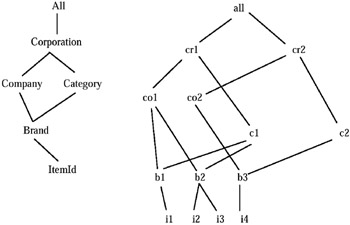Figure 29

### Unrelate Levels

The unrelate levels operator deletes a relation between two levels la and lb, such that la lb. The operator must guarantee that levels which are lower than la in the hierarchy will still be able to reach the same levels they reached before the unrelate operation. For instance, if la lb and lb lc, we must preserve la lc by making it explicit, in case we delete la lb, because, again, this relation was only implicit in the model.

### Delete Level

The delete level operator deletes a level and its roll-up functions. The level to be deleted cannot be the lowest one in the dimension (linf), unless it rolls-up to only one higher level. As was the case with the relate levels operator, taking into account that we only define the direct roll-ups, when deleting a level we must add the functions between levels above and below it.

The add instance operator inserts a new element, say x, into a level la (i.e., an element not belonging to the instance set of la). We must provide the operator with the pairs (li, xi), such that every li is a level to which la directly rolls-up (la li), and RUPlali (x) = xi), where RUP is a set of partial functions such that:

1. for each pair of levels l1, l2 such that l1 l2, there exists a roll-up function (partial function) RUPl1l2 : dom (l1) dom (l2);

2. for each pair of paths, in the graph with nodes in L and edges in , τ1 = <l1, l2, , ln-1, ln> and τ2 = <l1, l'2, , l'm-1, lm>, ln = lm, we have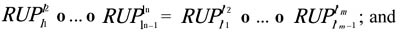3. for each triple of levels l1, l2, l3 L (with L a finite set of levels) such that l1 l2 and l2 l3,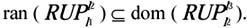### Delete Instance

The delete instance operator deletes an element belonging to the instance set of a level la. It is only defined when no element of any level li, such that l1 l2 rolls-up to the element being deleted.

An example of add instance and delete instance application is shown in Figure 30.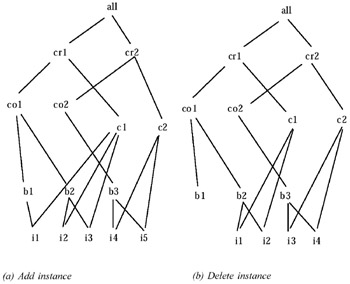Figure 30

Other operators, which explicitly refer to "levels" (insert, add, and delete level) and add multiplicity, proposed in Pourabbas & Rafanelli (2000), have been described in Chapter 4.Multidimensional Databases: Problems and Solutions
ISBN: 1591400538
EAN: 2147483647
Year: 2003
Pages: 150